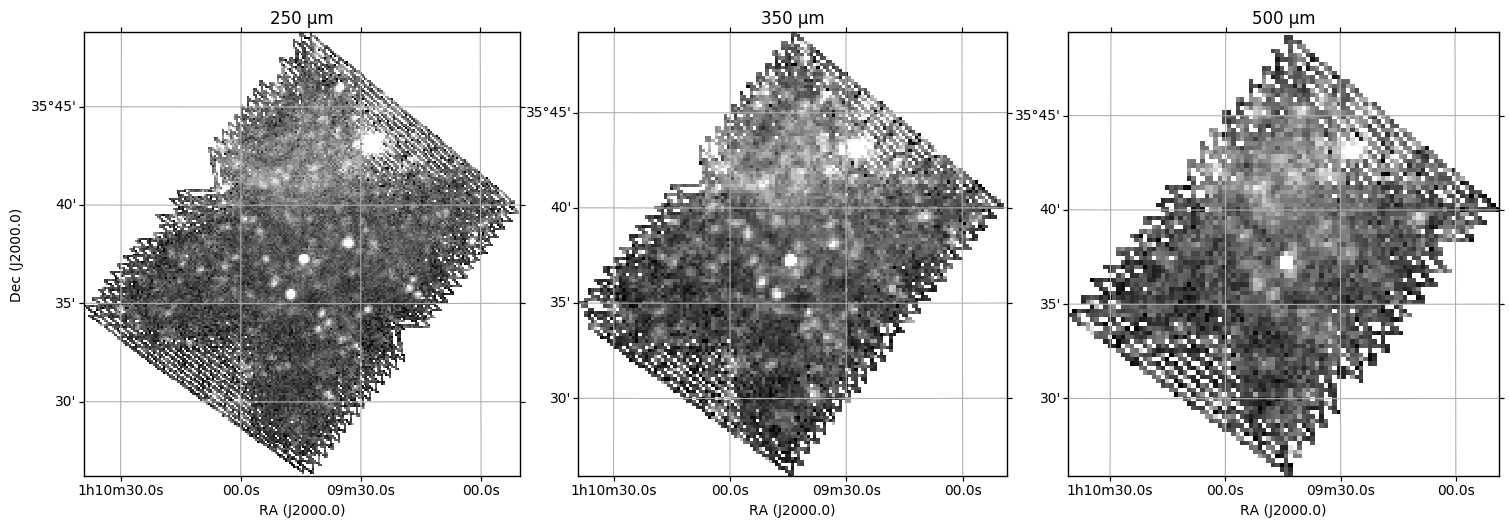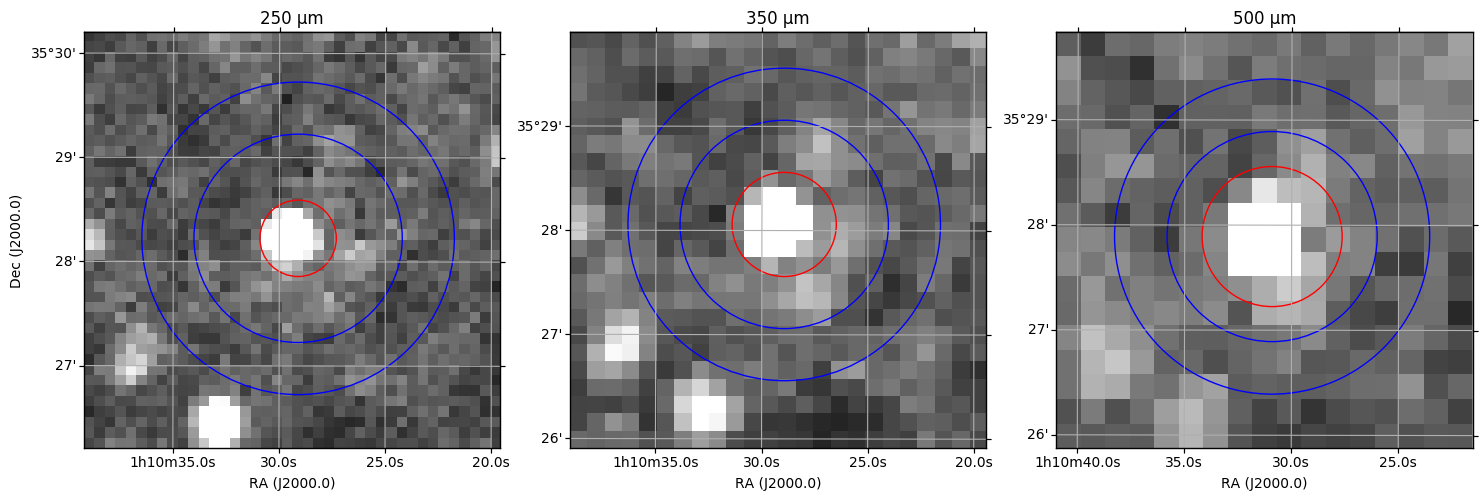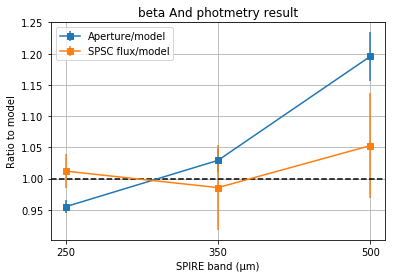# SPIRE Aperture Photometry for Point Sources¶

Author: Ivan Valtchanov

The method for photmetry is outlined in the SPIRE Handbook, section 5.12.1. This notebook just shows one practical example.

The workflow is the following:

1. Using ESASky module of astroquery search for the source in Herschel observations. Pick on observation for the example.
2. Download the map using the astroquery.esasky results.
3. Perform aperture photometry using photutils.
4. Bonus: compare the aperture-derived flux density with the one in the SPIRE point source catalogue, also available in astroquery.esasky.

For this exercise we are going to use a known stellar calibrator: $\beta$ And, with RA=01:09:43.9, Dec= +35:37:14.

For reference, the model flux densities of $\beta$ And in the SPIRE bands (Decin et al. 2007) are (430, 217, 105) mJy at (250, 350, 500) µm.

Requirements: python 3.x, matplotlib 2.2.2 (in matplotlib 3.0.0 the WCS from astropy 3.0.4 does not work), astropy, astroquery, photutils.

In :
import numpy as np
import os
# Set up matplotlib and use a nicer set of plot parameters
import matplotlib
import matplotlib.pyplot as plt
%matplotlib inline

In :
from astropy.io import fits
from astropy.wcs import WCS
from astropy.visualization import PercentileInterval, ImageNormalize
from astropy import units as u
from astropy.coordinates import SkyCoord
from astropy.table import hstack
from astropy.nddata import Cutout2D

from photutils import SkyCircularAperture, SkyCircularAnnulus, aperture_photometry

from astroquery.esasky import ESASky
from astroquery.simbad import Simbad

In :
#
# set the download dir for ESASky products
#
download_dir = os.path.expanduser('~') + "/tmp/" # change this to your desired directory
#
# define the target and get its coordinates from Simbad
target_name = 'beta And'
target = Simbad.query_object(target_name)
target_coord = SkyCoord(ra=target["RA"], dec=target["DEC"], unit=(u.hourangle,u.deg), frame='icrs')


## Search in ESASky¶

We search for Herschel maps in ESASky, using as an input the target name. The search radius is set to 6" (that's one pixel of the SPIRE 250 µm map). This should be sufficient.

In :
#
# We'll search ESASky for $\beta$ And directly, using the Simbad name resolver
maps = ESASky.query_region_maps(target_name, radius="6 arcsec",missions=['Herschel'])
#


## Check the ESASky results table¶

The results are returned in a astropy.Table.table. It is useful to check it.

In :
#
# let's check the results table and what observations are there
#
print (maps["HERSCHEL"].info)
maps["HERSCHEL"]["observation_id","filter","duration"].pprint()

<Table masked=True length=45>
name       dtype  format
--------------- ------- ------
postcard_url  object
product_url  object
observation_id  object
observation_oid   int32
ra_deg float64 {!r:>}
dec_deg float64 {!r:>}
target_name  object
instrument  object
filter  object
start_time  object
duration float64 {!r:>}
stc_s  object

observation_id     filter    duration
-------------- ------------- --------
1342224965 250, 350, 500    583.0
1342259256      100, 160    152.0
1342223338      100, 160    276.0
1342248031      100, 160    152.0
1342263815 250, 350, 500    583.0
1342248033      100, 160    276.0
1342223336       70, 160    276.0
1342237161      100, 160    276.0
1342212508       70, 160    276.0
1342199613      100, 160    276.0
...           ...      ...
1342237163       70, 160    152.0
1342223337      100, 160    152.0
1342237164       70, 160    276.0
1342223339      100, 160    276.0
1342248036       70, 160    276.0
1342199612      100, 160    276.0
1342248034       70, 160    152.0
1342199608       70, 160    152.0
1342237508 250, 350, 500    592.0
1342223334       70, 160    152.0
1342247980 250, 350, 500    583.0
Length = 45 rows

In :
# Let's pick up a SPIRE observation, i.e. one that has 250, 350, and 500 µm
# one example is 13422263815, this is index 4, we remove all the rest
nher = len(maps["HERSCHEL"])
ikeep = 4
maps["HERSCHEL"].remove_rows(np.delete(range(nher),ikeep))
maps["HERSCHEL"]["observation_id","filter","duration"].pprint()

observation_id     filter    duration
-------------- ------------- --------
1342263815 250, 350, 500    583.0

In :
# now we can download the map, we set the download_dir too
maps_data = ESASky.get_maps(maps,download_dir=download_dir)

Starting download of HERSCHEL data. (1 files)
Downloading Observation ID: 1342263815 from http://archives.esac.esa.int/hsa/whsa-tap-server/data?RETRIEVAL_TYPE=STANDALONE&observation_oid=8614128&DATA_RETRIEVAL_ORIGIN=UI [Done]
Downloading of HERSCHEL data complete.
INFO: Maps available at /Users/dbaines/tmp [astroquery.esasky.core]

In :
#
# extract the HDU for the maps in a dictionary
# there should be only one index 
hdu = {}
spire_bands = ['250','350','500']
for band in spire_bands:
hdu[band] = maps_data["HERSCHEL"][band]


## Visualise the maps¶

To visualise each SPIRE map we use the astropy.visualization.ImageNormalize module, doing a colour cut at the 98% of the pixel distribution, using the PercentileInterval. There are many options for pixel cut and stretch selection, so check the module documentation.

It is necessary to ignore the NaNs during the ImageNormalization.

Alternatively one can use APLpy for visualisation.

In :
#
# Display the three SPIRE maps, will use colour interval at 98%.
# NaNs are messing the normalization, so will take care of it
#
fig = plt.figure(figsize=(15,5),dpi=100)
pp = 98.0 #
for k,band in enumerate(spire_bands):
wcs = WCS(hdu[band]['image'].header)
ax = fig.add_subplot(1,3,k+1,projection=wcs)
ax.set_title(f'{band} µm')
lon = ax.coords['ra']
lon.set_axislabel('RA (J2000.0)')
lon.set_major_formatter('hh:mm:ss.s')
lat = ax.coords['dec']
if (k == 0):
lat.set_axislabel('Dec (J2000.0)')
else:
lat.set_axislabel('')
lat.set_major_formatter('dd:mm')
ximage = hdu[band]['image']
norm = ImageNormalize(ximage.data[~np.isnan(ximage.data)], interval=PercentileInterval(pp))
ax.imshow(ximage.data,norm=norm,cmap=plt.cm.gray,origin='lower',interpolation='nearest')
ax.grid(True)
plt.tight_layout(pad=2)## Converting the input maps from MJy/sr to Jy/pixel¶

To do aperture photometry we need to convert the SPIRE maps to Jy/pixel. The pipeline produced SPIRE maps are either in units of Jy/beam (point-source calibrated maps) or in MJy/sr (extended-source calibrated maps). The maps available in ESASky are the extended-source calibrated ones, so they are in units of MJy/sr.

Doing aperture photometry of a point source starting from the extended-source calibrated map will require the following steps, as explained in the SPIRE Handbook:

1. Convert to point-source calibration, dividing by KPtoE
2. Divide by $\Omega_\mathrm{pipe}$ to convert from per steradian to per pixel.

After these two steps the maps should be in Jy/pixel and we have to set the proper unit in the header.

In :
# check the map units, to make sure it's MJy/sr
print (hdu['250']['image'].header['BUNIT'])

MJy/sr

In :
#
# Using the SPIRE Handbook we copy the necessary parameters
# NOTE: we also apply the same scaling for the error image!
#
KPtoE = {'250': 90.646, '350': 51.181, '500': 23.580}
# the beam solid angle in arcsec^2
omega_pipe = {'250': 469.35, '350': 831.27, '500': 1804.31} # arcsec^2
for band in spire_bands:
wcs = WCS(hdu[band]['image'].header)
pixscale = wcs.wcs.cdelt*3600.0
hdu[band]['image'].data = pixscale**2*hdu[band]['image'].data/KPtoE[band]/omega_pipe[band]
hdu[band]['image'].header['BUNIT'] = 'Jy/pixel'
hdu[band]['error'].data = pixscale**2*hdu[band]['error'].data/KPtoE[band]/omega_pipe[band]
hdu[band]['error'].header['BUNIT'] = 'Jy/pixel'


Now the maps are in Jy/pixel. Let's define the coordinates of the target $\beta$ Andromeda:

And define the apertures for the target, and the background. We use the default aperture sizes as described in the SPIRE Handbook, table 5.8.

In :
aperture = dict()
aperture['250'] = SkyCircularAperture(target_coord, r=22. * u.arcsec)
aperture['350'] = SkyCircularAperture(target_coord, r=30. * u.arcsec)
aperture['500'] = SkyCircularAperture(target_coord, r=40. * u.arcsec)
# and the background annuli
back = SkyCircularAnnulus(target_coord, r_in = 60.0*u.arcsec, r_out=90.0*u.arcsec)


## Integrate the total flux¶

Next is the actual measurement of the total flux within the target aperture and the background annulus. We do the background subtraction within the loop over the bands.

We use the error extension of each SPIRE map and estimate the error on the derived flux within the input aperture.

A few comments:

• the aperture areas can only be calculated if the aperture is converted to pixels. To do this the method to_pixlel() needs a WCS.
• The final result is the flux density and the error, both in Jy.
In :
ap_flux = dict()
ap_flux_err = dict()
print ("band,flux,error")
for band in spire_bands:
#photo[band] = aperture_photometry(hdu[band]['image'], aperture[band])
img = hdu[band]['image'].data
wcs = WCS(hdu[band]['image'].header)
err_img = hdu[band]['error'].data
photo = aperture_photometry(img, aperture[band],error=err_img,wcs=wcs)
bkg_photo = aperture_photometry(img, back,wcs=wcs)
wcs = WCS(hdu[band]['image'].header)
bkg_area = back.to_pixel(wcs).area()
aper_area = aperture[band].to_pixel(wcs).area()
ap_flux[band] = photo['aperture_sum'] - aper_area*bkg_photo['aperture_sum']/bkg_area
ap_flux_err[band] = photo['aperture_sum_err']
print ("{},{:.4f},{:.4f} Jy".format(band,ap_flux[band],ap_flux_err[band]))

band,flux,error
250,0.3249,0.0036 Jy
350,0.1818,0.0032 Jy
500,0.0982,0.0032 Jy


## Corrections¶

For all the subsequent corrections we need the source spectrum, as the pipeline assumes a source with $\nu F_\nu$ = const. Our assumption is that in the SPIRE bands $\beta$ And has a powerlaw spectrum with an index 2 (i.e. Rayleigh-Jeans).

We can use the tables in the SPIRE Handbook and pick up the beam correction (Table 5.5) and the colour-correction for a point source (Table 5.7).

And finally, we apply the aperture correciton, which corrects for the point-like source flux lost outside the selected aperture. The corrections for the default apertures and sources with differnet spectral index, are listed in Table 5.9.

In :
# the beam correction
kbeam = {'250': 1.0446, '350': 1.0434, '500': 1.0746} # powerlaw with alpha=2
print ("band,flux,error")
for band in spire_bands:
ap_flux[band] = ap_flux[band]*kbeam[band]
ap_flux_err[band] = ap_flux_err[band]*kbeam[band]
print ("{},{:.4f},{:.4f} Jy".format(band,ap_flux[band],ap_flux_err[band]))

band,flux,error
250,0.3394,0.0038 Jy
350,0.1897,0.0034 Jy
500,0.1055,0.0034 Jy


Next we need to apply the colour correction KColP (Table 5.6)

In :
# the colour correction

kcolp = {'250': 0.9454, '350': 0.9481, '500': 0.9432} # powerlaw with alpha=2
print ("band,flux,error")
for band in spire_bands:
ap_flux[band] = ap_flux[band]*kcolp[band]
ap_flux_err[band] = ap_flux_err[band]*kcolp[band]
print ("{},{:.4f},{:.4f} Jy".format(band,ap_flux[band],ap_flux_err[band]))

band,flux,error
250,0.3209,0.0036 Jy
350,0.1798,0.0032 Jy
500,0.0996,0.0032 Jy


And finally the aperture correction, which is also source SED dependent (Table 5.8). Note that we use the standard apertures of (22,30,40) arcsec. For different ones the user has to use the beam profiles from the SPIRE calibration context and derive the correction.

In :
# the aperture correction

kaper = {'250': 1.28, '350': 1.242, '500': 1.2610}
print ("band,flux,error")
for band in spire_bands:
ap_flux[band] = ap_flux[band]*kaper[band]
ap_flux_err[band] = ap_flux_err[band]*kaper[band]
print ("{},{:.4f},{:.4f} Jy".format(band,ap_flux[band],ap_flux_err[band]))

band,flux,error
250,0.4107,0.0046 Jy
350,0.2233,0.0040 Jy
500,0.1255,0.0041 Jy


## Comparison with models¶

And finally let's compare with Decin et al. (2007) models:

In :
model = {'250': 430, '350': 217, '500': 105} # mJy
print ('band,measured,error,model,model/measured')
for band in spire_bands:
print ("{},{:.2f}+/-{:.2f},{},{:.2f}".format(band,ap_flux[band]*1000, ap_flux_err[band]*1000,model[band], model[band]/ap_flux[band]/1000.0))

band,measured,error,model,model/measured
250,410.72+/-4.60,430,1.05
350,223.35+/-3.98,217,0.97
500,125.53+/-4.07,105,0.84


We can visualise the apertures and the background annuli just to make sure they make sense.

We'll use Cutout2d to zoom in.

In :
fig = plt.figure(figsize=(15,5),dpi=100)
pp = 98.0 # colour cut percentage
zoomSize = u.Quantity((4.0,4.0 ), u.arcmin)
for k,band in enumerate(spire_bands):
wcs = WCS(hdu[band]['image'].header)
ax = fig.add_subplot(1,3,k+1,projection=wcs)
ax.set_title(f"{band} µm")
lon = ax.coords['ra']
lon.set_axislabel('RA (J2000.0)')
lon.set_major_formatter('hh:mm:ss.s')
lat = ax.coords['dec']
if (k == 0):
lat.set_axislabel('Dec (J2000.0)')
else:
lat.set_axislabel('')
lat.set_major_formatter('dd:mm')
ximage = hdu[band]['image']
norm = ImageNormalize(ximage.data[~np.isnan(ximage.data)], interval=PercentileInterval(pp))
cutout = Cutout2D(ximage.data, target_coord, zoomSize, wcs=wcs)
ax.imshow(cutout.data,norm=norm,cmap=plt.cm.gray,origin='lower',interpolation='nearest')
wcs_cut = cutout.wcs
aperture[band].to_pixel(wcs_cut).plot(ax=ax,color='r')
back.to_pixel(wcs_cut).plot(ax=ax,color='b')
ax.grid(True)
plt.tight_layout(pad=3)## Compare with the SPIRE Point Source Catalogue¶

The first release of the SPIRE Point Source Catalogue (SPSC) is available in ESASky, so we can extract the photometry in there and compare with the results from the aperture photometry.

In :
#
# search ESASky for $\beta$ And
#
# first, let's see what catalogues are available
ESASky.list_catalogs()

Out:
['AllWise',
'2MASS',
'INTEGRAL',
'CHANDRA',
'XMM-EPIC-STACK',
'XMM-EPIC',
'XMM-OM',
'XMM-SLEW',
'Tycho-2',
'Gaia DR2',
'Hipparcos-2',
'HSC',
'Herschel-HPPSC-070',
'Herschel-HPPSC-100',
'Herschel-HPPSC-160',
'Herschel-SPSC-250',
'Herschel-SPSC-350',
'Herschel-SPSC-500',
'Planck-PGCC2',
'Planck-PCCS2E-HFI',
'Planck-PCCS2-HFI',
'Planck-PCCS2-LFI',
'Planck-PSZ']
In :
#
# The following will extract the flux, its error and signal-to-noise ratio
#
# note the flux density is for a source with power-law SED with index $\alpha$ = -1.
#
# The SPSC is based on the timeline fitter, so it only needs the colour correction, see the Handbook, flowchart 5.21.
#
spsc_f = {}
spsc_f_err = {}
print ("band, Flux (mJy), Flux_err (mJy), SNR")
for band in ['250','350','500']:
output = ESASky.query_object_catalogs(target_name,f'Herschel-SPSC-{band}')
spsc_f[band] = output['flux']*kcolp[band]
spsc_f_err[band] = output['flux_err']*kcolp[band]
print ("{}, {:.2f}, {:.2f}, {}".format(band,spsc_f[band],spsc_f_err[band],output['snr']))

band, Flux (mJy), Flux_err (mJy), SNR
250, 435.17, 11.63, 37.4
350, 213.89, 14.70, 14.6
500, 110.54, 8.77, 12.5

In :
#
# let's see them graphically, we use the model as the baseline and do the ratio
#
spire_bands = ['250','350','500']
r1 = [] # aperture to model
r2 = [] # spsc to model
r1_err = [] # error aperture to model
r2_err = [] # error spsc to model
#
# will proagate the errors, assuming the model has zero error
#
for band in spire_bands:
r1.append(1000*ap_flux[band]/model[band])
r2.append(spsc_f[band]/model[band])
r1_err.append(1000*ap_flux_err[band]/model[band])
r2_err.append(spsc_f_err[band]/model[band])
#
#
fig = plt.figure(figsize=(8,5),dpi=100)
fig, ax = plt.subplots()
ax.axhline(y=1,color='k',ls='dashed')
ax.errorbar(spire_bands,r1,yerr=r1_err,marker='s',label='Aperture/model')
ax.errorbar(spire_bands,r2,yerr=r2_err,marker='s',label='SPSC flux/model')
ax.set_xlabel('SPIRE band (µm)')
ax.set_ylabel('Ratio to model')
ax.set_title(r'{} photmetry result'.format(target_name))
ax.grid(True)
ax.legend()

Out:
<matplotlib.legend.Legend at 0x11606c1d0>
<Figure size 800x500 with 0 Axes>